# Cats

Two cats caught two mice in two days. How many mouses will catch 6 cats for 6 days?

Correct result:

x =  18

#### Solution: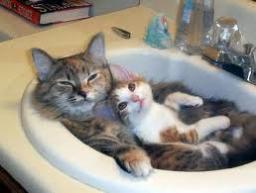Our examples were largely sent or created by pupils and students themselves. Therefore, we would be pleased if you could send us any errors you found, spelling mistakes, or rephasing the example. Thank you!

Leave us a comment of this math problem and its solution (i.e. if it is still somewhat unclear...):Be the first to comment!Tips to related online calculators
Check out our ratio calculator.

## Next similar math problems:

• A farmer's heritage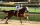A farmer died leaving his 17 horses to his 3 sons. When his sons opened up the Will it read: My eldest son should get 1/2 (half) of total horses; My middle son should be given 1/3rd (one-third) of the total horses; My youngest son should be given 1/9th (
• Four poplarsFour poplars are growing along the way. The distances between them are 35 m, 14 m, and 91 m. At least how many poplars need to be dropped to create the same spacing between the trees? How many meters will it be?
• Trees in alleyThere are four trees in the alley between which the distances are 35m, 15m and 95m. Trees must be laid in the spaces so that the distance is the same and the maximum. How many trees will they put in and what will be the distance between them?
• School year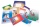At the beginning of the school year, 396 notebooks and 252 textbooks are ready to be distributed in the classroom. All pupils receive the same number of notebooks and the same amount of textbooks. How many pupils are there in the class if you know that th
• The lift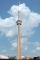The lift can fit 4 people. How many uphill rides must the lift make to move up 12 passengers?
• Twenty-fiveHow many three-digit natural numbers are divisible by 25?
• Twos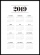Vojta started writing the number of this year 2019202020192020 into the workbook. .. And so he kept going. When he wrote 2020 digits, no longer enjoyed it. How many twos did he write?
• Collection of stampsJano, Rado, and Fero have created a collection of stamps in a ratio of 5: 6: 9. Two of them had 429 stamps together. How many stamps did their shared collection have?
• Bricks pyramidHow many 50cm x 32cm x 30cm brick needed to built a 272m x 272m x 278m pyramid?
• By sixFrom the digits 1,2,3,4 we create the long integer number 123412341234. .. .. , which will have 962 digits. Is this number divisible by 6?
• Three numbersWe have three different non-zero digits. We will create all 3 digits numbers from them to use all 3 figures in each number. We add all the created numbers, and we get the sum of 1554. What were the numbers?
• Sweets, candyGrandfather gave out sweets to four children. At the last moment, two more children came, so in order to have them all the same, each of the four children would receive four candies less than they would have received if they had not. How much did my grand
• Bdf tablet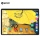Detective Harry Thomson has come across a surprising mystery. As part of the weekend's action, the tablet he looked for dropped by 30%. He also recommended this bargain to his friend. However, it came to the shop on Monday, and the tablet was 30% more exp
• Year 2020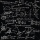The four-digit number divided by 2020 gives a result of 1, **. (Can not be in form 1,*0. ) Write all the options.
• Hens and pigs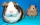Hens and pigs have 46 feet in total. At least how much can heads have?
• Reminder and quotientThere are given the number C = 281, D = 201. Find the highest natural number S so that the C:S, D:S are with the remainder of 1,
• Odd/even numberPick any number. If that number is even, divide it by 2. If it's odd, multiply it by 3 and add 1. Now repeat the process with your new number. If you keep going, you'll eventually end up at 1. Every time. Prove. ..